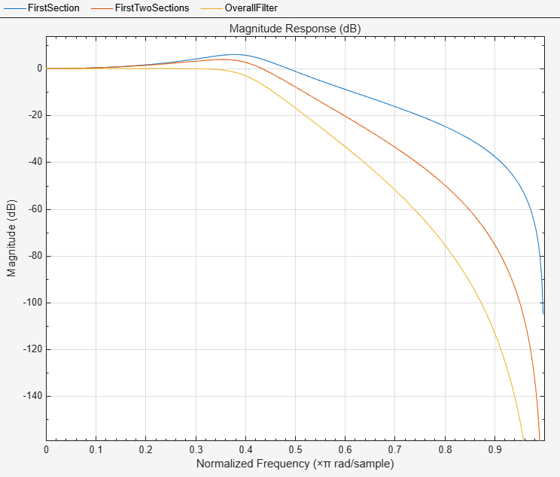# cumsec

Cumulative second-order section of the biquadratic filter

## Syntax

``sect = cumsec(sysobj)``
``sect = cumsec(sysobj,indices)``
``sect = cumsec(sysobj,indices,secondary)``
``cumsec(sysobj,...)``
``sect = cumsec(sysobj,'Arithmetic',arithType)``

## Description

example

````sect = cumsec(sysobj)` returns a cell array, `sect`, which contains cumulative sections of the biquadratic filter System object™, `sysobj`. Each element in `sect` is a filter with the structure of the original filter. The first element is the first filter section of the biquadratic filter. The second element of `sect` is a filter that represents the combination of the first and second sections of the biquadratic filter. The third element of `sect` is a filter which combines sections 1, 2, and 3 of the biquadratic filter. This pattern continues until the final element of `sect` contains all the sections of the biquadratic filter and should be identical to the biquadratic filter.```
````sect = cumsec(sysobj,indices)` returns the cumulative sections of the biquadratic filter object whose indices in the original filter are in the vector `indices`.```
````sect = cumsec(sysobj,indices,secondary)` uses the secondary scaling points `secondary` in the sections to determine where the sections should be split when `secondary` is `true`. `secondary` is `false` by default. This option only applies for biquadratic filter objects with `'Direct form II'` and ```'Direct form I transposed'``` structures. For these structures, the secondary scaling points refer to the location between the recursive and the nonrecursive part, that is the 'middle' of the section.```
````cumsec(sysobj,...)` plots the magnitude response of the cumulative sections using `fvtool`.```
````sect = cumsec(sysobj,'Arithmetic',arithType)` returns the cumulative sections of the biquadratic filter object with the arithmetic specified in `arithType`.```

## Examples

collapse all

This example plots the relative responses of the sections of a sixth-order filter with three sections. Each curve adds one more section to form the filter response.

```Lowpass = fdesign.lowpass('n,fc',6,.4); ButterLowpass = butter(Lowpass,'SystemObject',true); CumSections = cumsec(ButterLowpass); hfvt = fvtool(CumSections{1},CumSections{2},CumSections{3}); legend(hfvt,'First Section','First Two Sections','Overall Filter');```## Input Arguments

collapse all

Input filter, specified as one of the following System objects:

Filter indices. Use `indices` to specify the filter sections `cumsec` uses to compute the cumulative responses.

This option applies only when `sysobj` has the `df2sos` and `df1tsos` structures. For these second-order section structures, the secondary scaling points refer to the scaling locations between the recursive and the nonrecursive parts of the section (the "middle" of the section). Argument `secondary` accepts either `true` or `false`. By default, `secondary` is `false`.

Arithmetic used in the filter analysis, specified as `'double'`, `'single'`, or `'Fixed'`. When the arithmetic input is not specified and the filter System object is unlocked, the analysis tool assumes a double-precision filter. When the arithmetic input is not specified and the System object is locked, the function performs the analysis based on the data type of the locked input.

The `'Fixed'` value applies to filter System objects with fixed-point properties only.

When the `'Arithmetic'` input argument is specified as `'Fixed'` and the filter object has the data type of the coefficients set to `'Same word length as input'`, the arithmetic analysis depends on whether the System object is unlocked or locked.

• unlocked –– The analysis object function cannot determine the coefficients data type. The function assumes that the coefficients data type is signed, has a 16-bit word length, and is auto scaled. The function performs fixed-point analysis based on this assumption.

• locked –– When the input data type is `'double'` or `'single'`, the analysis object function cannot determine the coefficients data type. The function assumes that the data type of the coefficients is signed, has a 16-bit word length, and is auto scaled. The function performs fixed-point analysis based on this assumption.

To check if the System object is locked or unlocked, use the `isLocked` function.

When the arithmetic input is specified as `'Fixed'` and the filter object has the data type of the coefficients set to a custom numeric type, the object function performs fixed-point analysis based on the custom numeric data type.

### Topics

Introduced in R2011a

Watch now